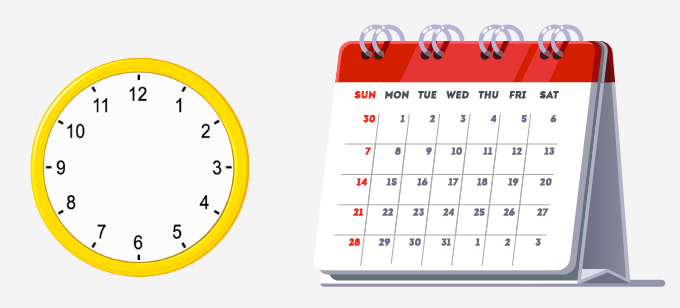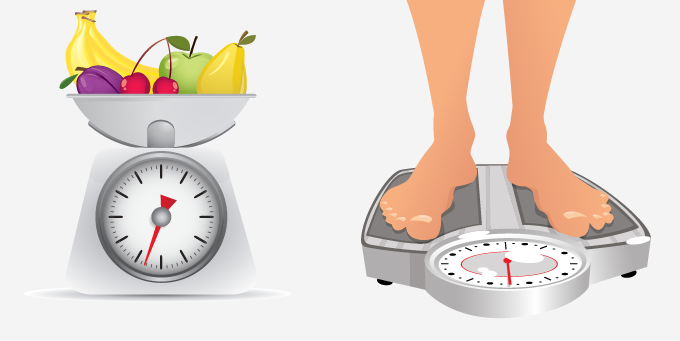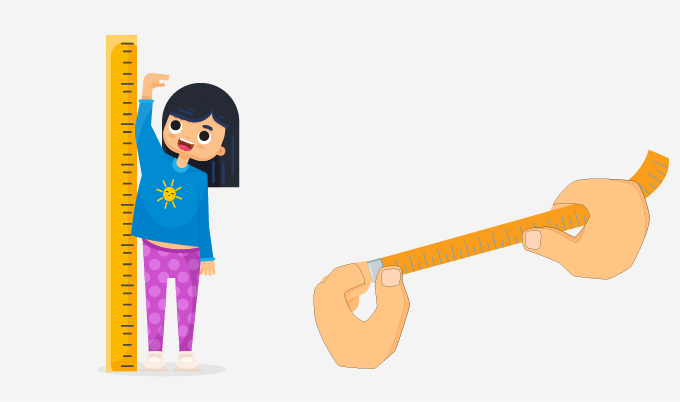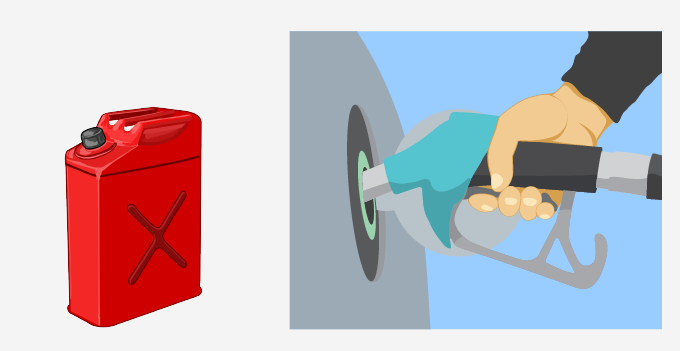# Measurement - Definition with Examples

The Complete K-5 Math Learning Program Built for Your Child

• 40 Million Kids

Loved by kids and parent worldwide

• 50,000 Schools

Trusted by teachers across schools

• Comprehensive Curriculum

Aligned to Common Core

## Measurement

• How old are you?
• How much do you weigh?
• How tall are you?
• How much water can be filled in your water bottle?
• How hot is it today?

To answer the above questions; what we need is to measure.

• To find how old you are, you need to measure time.
• To know how much you weigh, you must weigh yourself.
• To know how tall you are, you need to measure your height (length)
• To know how much water you can fill in your water bottle, you need to measure the capacity of your bottle.
• To find out how hot it is today, you need to measure the temperature.

So, what exactly is “measurement”?

Measurement is a process of finding a number that shows the amount of something.

## Time

The ongoing sequence of events is time. We can measure time in seconds, minutes, hours, days, weeks, months, and years.A clock and a calendar help us to measure time.

## Weight

The amount of matter a thing consists of is called its weight. Measuring weight means to measure the heaviness of a thing.Weight can be measured in grams, kilograms, and pounds.

## Length

The amount of something that is measured from one end to the other along the longest side is called its length.Length is measured in centimeters, meters, kilometers, feet, and miles

## Capacity

Capacity is a measure of how much quantity a thing can hold.Capacity is measured in liters and gallons

## Temperature

The temperature of a thing is the measurement of how hot or cold it is.Temperature is measured in Celsius, Fahrenheit, and Kelvin.

We can convert from one unit of measurement to another.

There are two measurement systems:438834709

The Metric System:

This system is based on the meter, liter, and gram as units of length (distance), capacity (volume), and weight (mass) respectively.

The US Standard Units:

This system uses inches, feet, yards, and miles to measure length or distance.

Capacity or volume is measured in fluid ounces, cups, pints, quarts or gallons.

Weight or mass is measured in ounces, pounds and tons.

 Fun Facts: The metric system was first proposed by the French astronomer and mathematician Gabriel Mouton in 1670 and was standardized in Republican France in the 1790s. The US Standard units are also known as "English Units" or "US Customary Units".

Won Numerous Awards & Honors